# Professor Jang Again Tortures CSP Mathematics Until it Breaks

In which my life is made a little harder.

We first met Professor Jang in a “Comments on the Literature” type of post from 2016. In that post, I pointed out fundamental mathematical errors contained in a paper the Professor published in the IEEE Communications Letters in 2014 (The Literature [R71]).

I have just noticed a new paper by Professor Jang, published in the journal IEEE Access, which is a peer-reviewed journal, like the Communications Letters. This new paper is titled “Simultaneous Power Harvesting and Cyclostationary Spectrum Sensing in Cognitive Radios” (The Literature [R144]). Many of the same errors are present in this paper. In fact, the beginning of the paper, and the exposition on cyclostationary signal processing is nearly the same as in The Literature [R71].

Let’s take a look.

It’s important to look closely at the CSP mathematics in this paper, and in the first one, because it is now a pattern. Not only does Professor Jang have serious problems with the basic mathematics of CSP, but so do multiple reviewers and editors. (That is the most charitable interpretation I can muster for the reviewers.)

### Jang’s Exposition

The Professor starts off just fine:I could quibble with the equality between (2) and (3) being “shown” in an Appendix: it is just a consequence of assumed cycloergodicity. But that is a small issue here. Equation (5) is indeed the cyclic periodogram, which reduces to the conventional periodogram when$\alpha = 0$. The exposition does not deal with the conjugate parameters, which is consistent with later sections of the paper where the various PSK modulated signals are all real-valued.

The Professor continues:Equation (7) is fine–the spectral correlation function and the cyclic autocorrelation function are a Fourier transform pair. However, Equation (8) is not true, and the mathematical problems have started. The limit in (8) does not exist for random power signals, such as all common models of PSK, CPM, DSSS, FH, OFDM, etc. signals. The lack of convergence is well-known in the case of$\alpha=0$. This is why all periodogram-based non-parametric spectrum estimators apply some kind of averaging (temporal or spectral smoothing or both) to the periodogram in order to estimate the power spectrum. Similarly for the cyclic periodogram and the spectral correlation function: see what I call the frequency-smoothing and the time-smoothing methods of spectral correlation estimation, which are based on the cyclic periodogram.

The Professor now introduces the weak-signal maximum-likelihood detector for a cyclostationary signal in white Gaussian noise (see My Papers ):Equation (11) is OK, and it reduces to (12) when the signal$x(t)$ is present and is corrupted by stationary noise, and the observation interval for the detection problem extends to infinity. Which is fine. Equation (13) is OK too, it just defines an individual component of the sum in (12).

Continuing on, the Professor wishes to somehow simplify (12) so we don’t have to estimate a whole lot of spectral correlation function slices and integrate their squared magnitudes.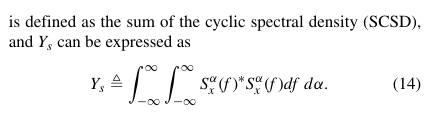Here is another major misstep. We know that modulated signals exhibit at most a countably infinite number of cycle frequencies, but most often just exhibit a finite (and small) number. Rectangular-pulse PSK/QAM exhibits a countably infinite number, whereas square-root raised-cosine PSK/QAM exhibits only a handful. Even high-processing-gain DSSS exhibits only hundreds or low thousands of cycle frequencies. That is, the spectral correlation function is discontinuous in the cycle-frequency parameter, and for any slice through the spectral correlation function with spectral frequency$f$ held constant, the slice is non-zero only at a few isolated points, or at most an infinite set that has measure zero over the real numbers specifying$\alpha$. This just boils down to the fact that the integral in (14) is almost always zero. An exception can happen for signals with impulsive spectral correlation functions, which are not considered in the Professor’s paper.

Jang continues: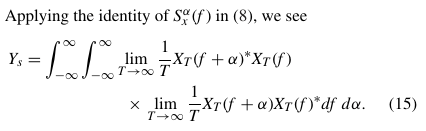The false (8) is substituted in the false (14) incorrectly here. You’d really want to use two different limits with independent$T$ values, such as$T_1$ and$T_2$. In any case, though, the limit of the cyclic periodogram is not the spectral correlation function, so the substitution is invalid.

Having written the double integral over the product of spectral correlation functions, then expressing the spectral correlation functions as limits of cyclic periodograms, the Professor now tries to identify those same cyclic periodograms as conventional periodograms … somehow.Here is where the lack of using separate$T_1$ and$T_2$ variables in the two limits allows the Professor to blithely regroup terms and try to reapply the limits to the regrouped terms. So now$Y_s$, which is (12), the value of the detection statistic for an infinite interval on the signal-present hypothesis, which depends on the spectral correlation function for the signal to be detected, depends only on the power spectrum of that signal$S_x(f)$. Cyclostationarity has been reduced to stationarity.

Digging the hole deeper:But, wait, Equations (12) and (13) imply$\displaystyle Y_s = \sum_\alpha Z(\alpha) \hfill (A)$

and (17) is$\displaystyle Y_s = \int_{-\infty}^\infty S_x(\alpha) \otimes S_x(-\alpha)^* \, d\alpha \hfill (17)$

How can (A) and (17) both be true?

The Professor turns to$Z(\alpha)$ and concludesNow,$Z(\alpha)$ is perfectly well defined in (13) and differently but perfectly well defined in (18). Equation (13) together with basic properties of the spectral correlation function implies that$Z(\alpha)$ is a bounded function that is non-zero only on a set of measure zero in the real numbers defining$\alpha$. Equation (18) together with knowledge of typical PSDs for communication signals (not controversial) implies$Z(\alpha)$ is a bounded function with its energy either completely contained in a finite interval (square-root raised-cosine PSK/QAM) or is an energy signal (rectangular PSK/QAM).

But the right hand side of (19) is a power signal. It is periodic with period$T_0$, and so can be expressed as a Fourier series, and therefore it has a Fourier transform that consists of a set of impulse functions.

Therefore$Z(\alpha)$ (either definition) and the RHS of (19) cannot be a Fourier transform pair.

Finally, the CSP exposition ends with the following wrap-up: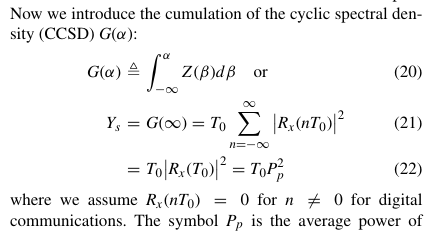The conclusion is that$Y_s$, originally defined in (12) and then changed to something different in (17), depends only on the symbol rate of the signal and on the power of the signal.  This conclusion is based on a sequence of false mathematical steps, and cannot be true in any case. We can exhibit a set of random processes corresponding to communication signals that have exactly the same power$P_p$ and symbol rate$1/T_0$ but that have different$Y_s$ values as defined by (12). And remember, though it may be difficult to keep all these mistakes straight, that (12) is true and useful.

### Illustrative Example

Professor Jang considers real-valued rectangular-pulse BPSK, QPSK, OQPSK, and also MSK, which is OQPSK with pulses equal to half-periods of sine waves (not controversial; well-known My Papers ). Let’s look at the first three in detail. Suppose they each have identical average power$P_p = 1$ ($0$ dB), identical symbol rates$1/T0 = 1/10$, identical carrier frequencies$f_c = 0.25$, and identical rectangular pulse functions. So, these signals will have identical autocorrelation functions,Figure 1. Autocorrelation functions for three real-valued rectangular-pulse signals of interest to Professor Jang. Note that they are identical.

and identical power spectra,Figure 2. Power spectral density estimates for three real-valued rectangular-pulse signals of interest to the Professor. They are identical, which is consistent with their ideal mathematical formulas.

So from Jang’s (18) or (22), these three signals will have identical$Z(\alpha)$ and identical$Y_s$. But in reality they have very different sets of cycle frequencies for which their spectral correlation function is non-zero. The cycle-frequency sets are$\alpha_{BPSK} = \{k/T_0\} \cup \{2f_c + k/T_0\} \cup \{-2f_c + k/T_0\} \hfill (B)$$\alpha_{QPSK} = \{k/T_0\} \hfill (C)$$\alpha_{SQPSK} = \{2k/T_0\} \cup \{2f_c + (2k+1)/T_0\} \cup \{-2f_c + (2k+1)/T_0\} \hfill (D)$

for all integers$k$. However, the spectral correlation function is only significant (relative to the signal’s average power$P_p$) for$|k| < 4$ or so.

The right hand side of (19) is identical for the three signals: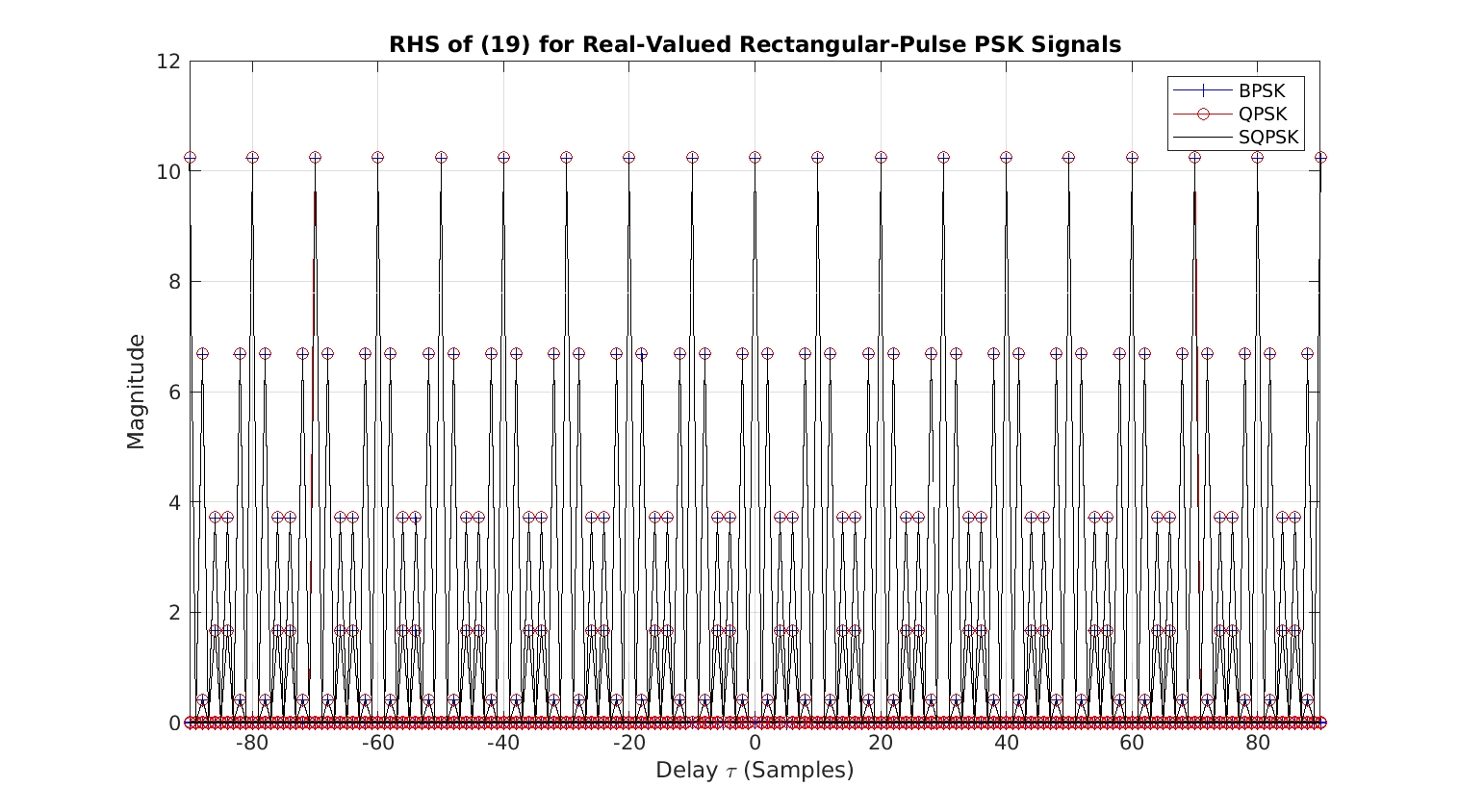Figure 3. The right-hand side of (19) in the discussed paper, evaluated for three real-valued rectangular-pulse PSK signals of interest to the professor. Note that the three evaluations of the right-hand side of (19) are identical.

But the$Z(\alpha)$ functions from (13) are highly distinct: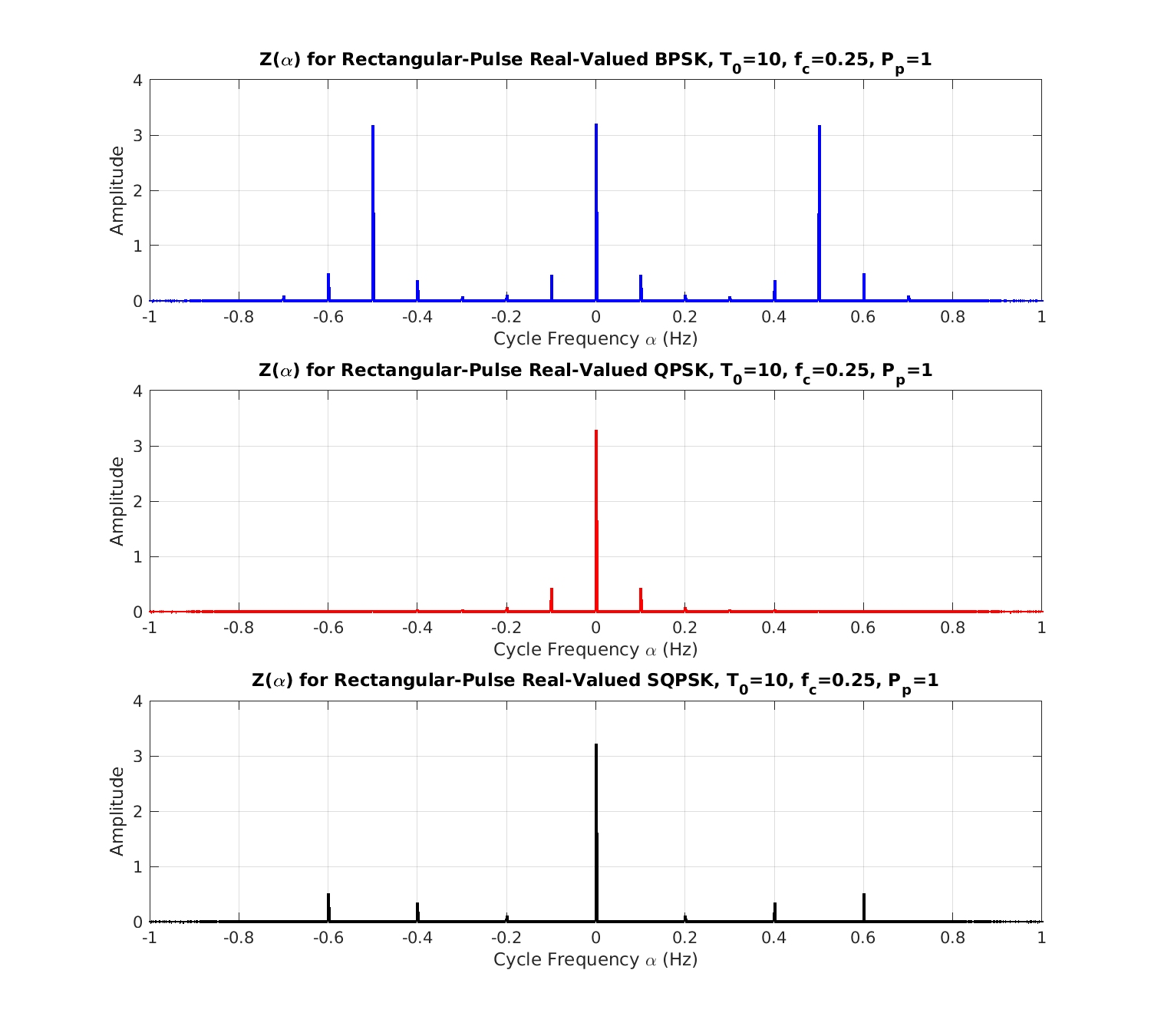Figure 4. Evaluation of from (13) for three real-valued rectangular-pulse PSK signals of interest to the professor. Note that they are distinct, contradicting (19).

This implies that the Fourier transform pair relation in (19) cannot be true.

The true values of$Y_s$ are 13.3 for BPSK, 5.4 for SQPSK, and 4.6 for QPSK. So (18) cannot be true: you cannot reduce the multi-cycle detector output to a function of the PSD. Signals with identical PSDs can have very different cycle-frequency patterns and amounts of cyclostationarity!

### What’s Going on Here?

The publication pattern is bigger than I let on in the introduction to this post because I’ve also participated in the peer review of a third paper from Professor Jang, and that paper contained many of the same basic mathematical errors as we’ve seen in The Literature [R71] and now in The Literature [R144]. Assuming the Professor reads the CSP Blog would be hubris. But I know for a fact that these refutations have been supplied to the Professor, and now I also know that they have been ignored. And the Professor’s continuing ability to do that must be due to the reviewers and editors involved in each of these submissions. Or … I’m wrong about something here. You be the judge, and let me know.I'm a signal processing researcher specializing in cyclostationary signal processing (CSP) for communication signals. I hope to use this blog to help others with their cyclo-projects and to learn more about how CSP is being used and extended worldwide.

## 2 thoughts on “Professor Jang Again Tortures CSP Mathematics Until it Breaks”

1.Dylan Gormley says:

Hey, Chad. I hope this reaches you in good spirits. I’m writing my master’s thesis right now on a cyclostationary feature detection implementation. I’ve read a few of these posts where you grill people and I really don’t want to be that guy… ha. It’s my first publication, and it’s important to me to not produce poor work. I’ve spent a lot of time reading Van Trees and this blog. If you have any time would you mind giving it a read and giving me your criticism?

1.Chad Spooner says:

Thanks for stopping by the CSP Blog Dylan! I appreciate it.

Sure, I can take a look. I’ll connect with you via email. The fact that you mentioned Van Trees is promising!